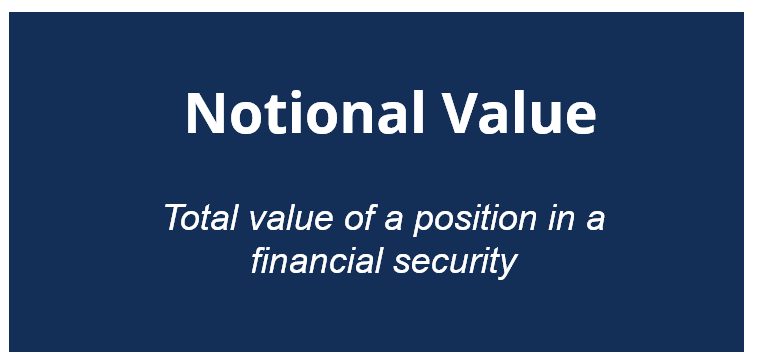# Notional Value

The total value of a position in a financial security

## What is Notional Value?

Notional value (also known as notional amount or notional principal amount) is the face value on which the calculations of payments on a financial instrument (e.g., swap) are determined. In other words, the notional amount indicates how much money is controlled by a position on a particular financial instrument.The notional value is quoted for different derivatives such as swaps, equity options, and futures. It generally used to distinguish the total value of a position from the total cost required to obtain the position.

### Notional Value vs. Market Value

The concept of notional value must not be confused with the concept of market value. Although both terms describe the value of a financial security, the concepts are used in different contexts.

Notional value is the total value of a position in a financial security. Strictly speaking, it determines how much money a position in securities control. In other words, the value can be viewed as a theoretical value of a position in securities.

Conversely, market value indicates the marketplace value of security set by the buyers and sellers. Essentially, it is the price of financial security at which it can be bought or sold. Therefore, the market value is an actual value of a position in securities.

### Uses in Interest Rate Swaps

Interest rate swaps are swaps in which the counterparties agree to exchange future interest payments. The interest payments are calculated on the predetermined notional principal value. The notional value can be denominated in any currency and can take any value.

The payable interest rate payments are calculated periodically by multiplying the appropriate interest rates by the notional principal value. Strictly speaking, the notional principal value in interest rate swaps is a purely theoretical value that is employed only for the calculation of interest payments.

### Uses in Currency Swaps

Currency swaps can be considered as a type of the interest rate swaps in which the counterparties exchange the principal and interest payments denominated in different currencies (e.g., US dollar vs. British pound). Similar to interest rate swaps, the calculation of interest rate payments for the currency swaps is also based on the predetermined notional principal values.

Note that currency swaps involve two notional values denominated in two different currencies. Unlike interest rate swaps, currency swaps also involve the exchange of notional principal values.

### Uses in Equity Options

Equity options (such as calls or puts) provide the right but not the obligation to buy or sell the underlying shares at the specified price at some point in the future. Commonly, each option provides the opportunity to buy or sell 100 shares. The nominal value for equity options is the total value of a position in the options an investor holds.

In other words, the nominal value for an option can be determined by multiplying the number of underlying shares by the strike price of a share. For example, if a call option provides to its holder the right to obtain 100 underlying shares at \$15 per share, the nominal value of this option is \$1,500 (\$15 x 100).

### Additional Resources

CFI is the official provider of the global Financial Modeling & Valuation Analyst (FMVA)™ certification program, designed to help anyone become a world-class financial analyst. To keep advancing your career, the additional resources below will be useful:

• Embedded Option
• Financial Intermediary
• Secondary Market
• Trading Mechanisms

### Valuation Techniques

Learn the most important valuation techniques in CFI’s Business Valuation course!

Step by step instruction on how the professionals on Wall Street value a company.

Learn valuation the easy way with templates and step by step instruction!